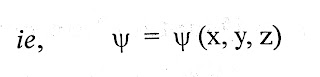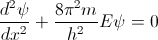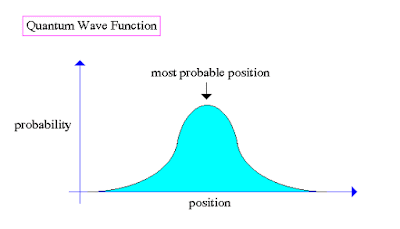## Header Ads

Scientists of early 20th century tried to explain the behaviour of atom on the basis of dual nature of matter and uncertainty principle. They had to develop a new view of matter and energy to accurately describe how atoms behaved. This attempt is called Wave Mechanics or Quantum Mechanics. This theory describes matter as, acting both as a particle and as a wave. In case of visible objects in every day life the wave length is too small to be apparent. Wave like nature becomes important in microscopic particles such as electrons. Due to the wave nature of electrons they exist as a fizzy cloud of negative charge around the nucleus, instead of as a particle located at a single point.

In 1926, Erwin Schrodinger suggested an equation to describe the movement of electron in an atom. The equation is known as Schrodinger Wave Equation (SWE) and it is written as:are the second differential coefficient of ψ with respect to x,y and z, E is the total energy of electron, 'm' is the mass of electron and ψ (psi) is the wave function.

An electron in an atom is described as a wave. A wave can be recognized by its position in space and its amplitude at that the point. The wave function 'ψ' represented the amplitude of wave. The amplitude ψ is thus a function of space co-ordinates and time. At any particular instant ψ is a function of space co-ordinates only.The wave function itself has no physical significance. But square of wave function (ψ2) has some physical significance. For an electronic wave function, ψ2 at any point is proportional to the probability of finding electron at that point.

So last time we looked at the corner stone of Quantum Mechanics - Schrodinger equation:

* Note it's a simplified version for 1 dimension.

This time we're gonna look at some simple solutions of this equation, which will show us how microscopic particles behave.

So let's start by simplifying Schrodinger equation for a case of a free moving particle. Since the zero of potential is an arbitrary choice we choose to make U0 = 0. This gives:(1)

Also it is useful for our solution to express the energy E in terms of momentum p (where Ek= (mv^2)/2 & p=mv), which gives:

Substituting (2) into (1) gives:

This can be simplified further using De Broglie's wavelenght (something we covered last time: p=h/lambda) and defining a quantity called wave number k: k= 2pi/lambda:

( Schrodinger equation for a free moving particle)

So how can we solve this equation? We'll it's not a trivial process, and it doesn't really matter for learning the basics, so let's skip to the answer:

(where A is some constant, e is the exponential function, i is the square root of -1 from complex numbers, k is the wave number)

Note that this is a time-independent solution. The time dependence of the wave function comes from solving the time dependent Schrodinger equation, which gives:

SE for a free moving particle:

Solution for this equation:

So what do these equations tell us about the free moving particle? To find the answer to this question we need to find the probability density function. If you recall, the probability density function is basically just the square of an absolute value of the wave function. So by calculating the probability density function we would get:

|Psi |^2 = Psi x complex conjugate of Psi =
= A(cos(kx − wt) + i sin(kx − wt))A(cos(kx − wt) − i sin(kx − wt))
= A^2(cos2(kx − wt) + sin2(kx − wt))
= A^2

Now if you look at the result more carefully, you should realise that there's some strange stuff going on. The probability density function is constant, that means that the probability of finding a the particle in any point of space is equal. In more simple words, you have the same probability of finding the free moving particle in your room and somewhere in the other side of the universe. This is due to the fact that we have not taken into account the uncertainty of the momentum of a particle.So you might be asking  - why are we studying free moving particles, while in reality most of the matter is situated in confined atoms and molecules. And you're definitely right, so let's look at another system, which might help us understand the behaviour of electrons, which are "trapped" in an orbit of an atom.

The easiest way of understanding simple atoms or similar systems is using a thing called infinite potential well. That is a system in which a particle is trapped between infinite potential "walls", which can be for instance be various electric of magnetic fields or anything similar, which confines the particle in a given space. After all, in a sense electrons orbiting a nucleus are also in a similar potential well - if they get too close to the nucleus, they are pushed back, if the get too far they are attracted back.

Infinite potential well:

So how does particle behave in such a well? Well let's firstly imagine what would happen in a classical situation, let's say for a tennis ball trapped between two walls. It's clear that (neglecting gravity, which would eventually bring the ball down) any position between the walls has the same probability of finding the ball there.

However, in quantum mechanics, things are different. We already know that particles tend to behave as probability waves, so it's clear that the probability of finding the particle in any point of space will not be equal( a good analogy of a wave in a sort of potential well is a rope fixed at two places and oscillating).

So how can we find out at which points of space we are most likely to find the particle? By solving the Schrodinger equation of course.

And since this is a basic course we're not gonna bother ourselves with the process of solving the SE. We're just gonna skip right to the solution which is:

Wave function for a particle confined in a infinite potential well: Psi(x) = Asin kx

Now to calculate the probability density function, we need to apply the initial conditions of the system - as we know the particle can't be in points of space, which are beyond the boundaries.

In an infinite potential well the boundary conditions imply:
Psi (0) = Psi  (L) = 0.
Resulting in the limitation that k must have one of the discrete set of values
kn = (pi . n)/L , where
n = 1, 2, 3 . . .

Now when we know k, we can find allowed energies.
Using  E= (p^2)/m and De Broglies wavelength p= h/ lambda = hk/ 2pi gives the following:

En = (h^2 . n^2)/(8mL^2)
n = 1, 2, 3 . . .

The allowed energies for the trapped particle are quantised according to the value of
n which is known as a quantum number.

Note that we can calculate the quantum number using simple calculations, which we'll look at later. It's important to notice that for macroscopic objects n is very larger, which gives rise to the so called correspondence principle - the idea that if n is very large (which is definately the case for macroscopic objects), quantum mechanics prediction become similar to classical physics prediction.

And we can see this by finding the probability function of the infinite potential well, and graphing it with different values of n:

As we can see as n grows, the wavelenght of the wave in the graph becomes shorter. In n was very very big, it would look as every point in the confined space has the same probability (like in the classical physics case).